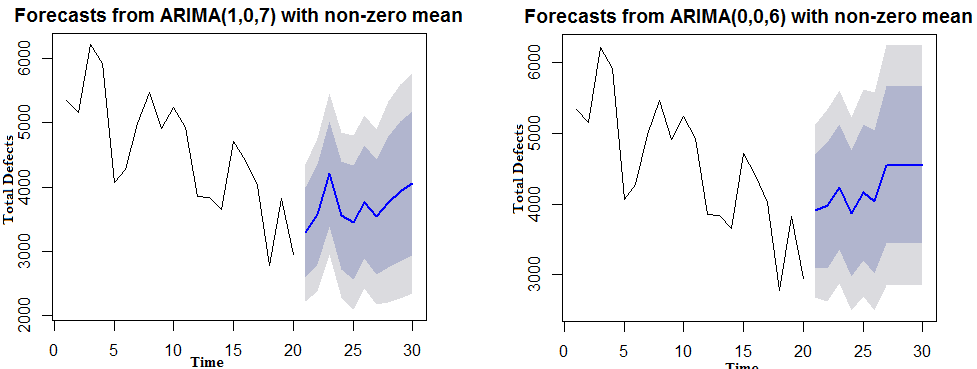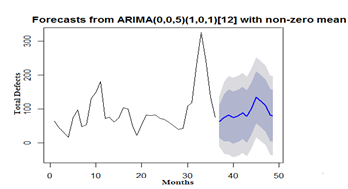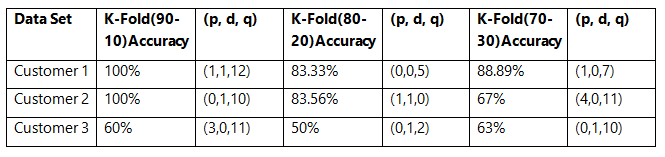Time Series-based Forecasting using ARIMA Models | HCLTech

# Time Series-based Forecasting using ARIMA Models

View Tags

## Time Series-based Forecasting using ARIMA Models April 01, 2015### Introduction

ARIMA uses regression analysis, the purpose of which is to predict future points. Lags of the Stationary Series in the forecasting equation are defined as “autoregressive” (AR) terms. Lags within the Forecast Series are called “moving average” (MA) terms. The Time Series which turns non-stationary data in to stationary by applying the difference is called “integrated” (I).

The main challenge with the Holt method is the use of a single parameter-based forecast. Whereas, ARIMA calculates trends, seasonality, errors, and stationary and non-stationary series of data sets when forecasting data.

ARIMA

The main objective of the ARIMA model is for forecasting (predicting future values of the Time Series). The model is generally referred to as ARIMA (p, d, q), where p, d and q are non-negative numerical values.

These three parameters are defined as follows:

“p” stands for  autoregressive terms

“d” stands for number of seasonal and non-seasonal differences

“q” stands for forecast errors (or) moving average terms

ARIMA models are defined for stationary Time Series. If our Time Series object is non-stationary, then we will have to obtain the Stationary Series data from the non-Stationary Series by applying the ‘difference’ (d), where ‘d’ refers to the order of differencing.

To find the accurate value with max %, there are diagnostic tests to try and find the best fit values of (p, d, q).

Illustration – The defects log forecast for the future 12 months based on past 3 years data using ARIMA and SARIMA (Seasonal ARIMA).In the above graph, when parameters (AR (p) changes to ‘1’, and then to ‘0’, the regression too has changed and the Moving Average (MA) q value has changed from ‘6’ to ‘7’. The main challenge here is to find which regression parameter is suitable to fit a model with more than 90% accuracy.

Seasonality with ARIMA (SARIMA)

Seasonal ARIMA is the model in which a pattern repeats seasonally over time. In addition to the non-seasonal parameters, seasonal parameters for a specified interval (recognized in the identification phase) need to be estimated. Similar to the simple ARIMA parameters, there are: seasonal autoregressive (ps), seasonal differencing (ds), and seasonal moving average parameters (qs).The seasonal ARIMA model combines both non-seasonal and seasonal factors in a multiplicative model.

ARIMA (p, d, q) × (P, D, Q) S,

Where, p = non-seasonal AR order

d = non-seasonal differencing

q = non-seasonal MA order

P = seasonal AR order

D = seasonal differencing

Q = seasonal MA order

S = time span of repeating seasonal patternK-fold is the technique to divide the data into ‘k’ subsets as training and testing data sets for computing accuracy. The pattern below shows cross-validation using K-fold accuracy for different (p, d, q) values of “Defects Logged Data”.

* +/- 10% of the predicted difference is deemed acceptable.